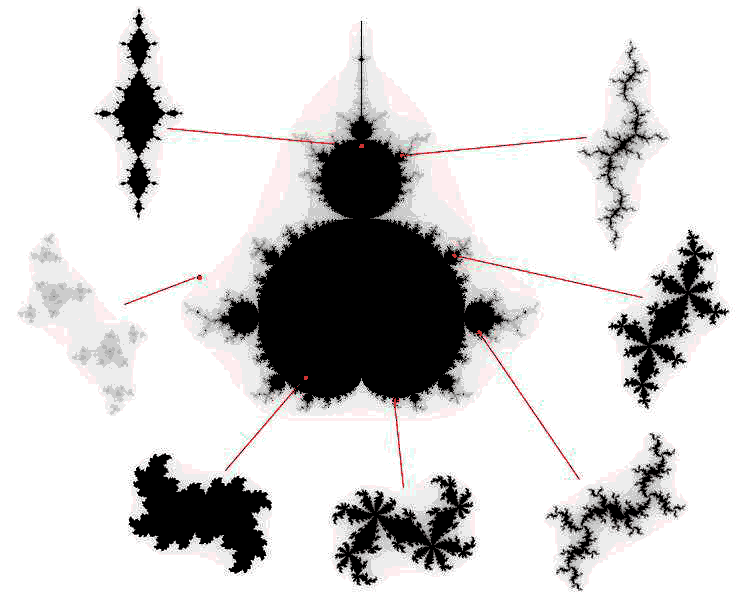Solutions

Exercises:

# Introduction

In this session, we study fractals generated by means of "escape-time" algorithms. Such fractals are computed by repeatedly applying a transformation to a given point in the plane. The obtained series of transformed points is called the orbit of the initial point. An orbit diverges when its points grow further apart without bounds. A fractal can then be defined as the set of points whose orbit does not diverge.

Consider the transformation z' = z2 in the complex plane C. Recursively applying this formula to a complex point in the plane z generates orbits that converge to 0 when |z|<1, diverge when |z|>1, and follow a perfect circle when |z|=1.

The orbits of the fractal generated by the transformation z' = z2 are easy to predict. Things become much more complicated when adding an arbitrary constant to the transformation function. That is, consider the transformation z' = z2 + c (where c is any complex number). For such a transformation, it is impossible to predict for which points their orbit will diverge or not. We can, however, plot (an approximation of) the resulting fractal by using the brute force computing power of our PC to calculate the orbits and check whether they (seem to) diverge.

You can generate a fractal by calculating the orbit for each point in the plane (that is: pixel on the screen) and checking whether it diverges. You can generate a black-and-white image of the fractal by colouring a pixel white if its orbit diverges, and black if its orbit does not. However, you can also create (much prettier) coloured images by making the color of a pixel correspond to how fast its orbit diverges. That is, the colour of the pixel corresponds to the number of transformations that were required to make the orbit diverge (i.e. escape from a predefined bounding box or circle centered around the point). By using a coloured image, you will see how 'fast' points along the sides of the fractal diverge. You can use the following source code to start from.

# Exercises

## Exercise 1: the Julia Fractal

The "filled-in" Julia set (after the French mathematician Gaston Julia) is the set of all points in the plane whose orbit does not diverge. For the transformation z' = z2, the Julia set corresponds to a filled circle. Draw the "filled-in" Julia sets for the following transformations:

• z' = z2 - 1
• z' = z2 - 2
• z' = z2 + 0.2 + 0.3i
• z' = z2 + i

For each pixel on the screen, treat it as a complex number by mapping the screen coordinates to the complex plane, such that the pixel (w/2, h/2) corresponds to the origin (the complex number 0 + 0i). Then recursively apply one of the above transformations to the point and check whether this series diverges. An easy approximation to check whether the series diverges is to see whether the transformed point lies outside of a circle with radius 3 centered around the origin. If the transformed point still does not fall outside of this circle after about 30 iterations, you can conclude that the orbit converges and the point belongs to the fractal.

Once you can draw a Julia fractal, try animating your fractal by changing the value of the constant c for each animation frame.

## Exercise 2: the Mandelbrot Fractal

The Mandelbrot fractal (after the mathematician Benoit Mandelbrot) is probably the most famous fractal. There exists a nice relationship between this fractal and the Julia fractal from Exercise 1. As you probably noticed, the shape of each Julia set changed dramatically based on the constant c. Some Julia sets could be depicted as a single shape (mathematically speaking: it is a connected set) while others were depicted more like a cloud of points (mathematically: a disconnected set). The Mandelbrot set is defined as the set of complex numbers c for which the Julia set z2 + c is connected. You can consider the Mandelbrot set as a kind of "atlas" of Julia sets, each point in the Mandelbrot fractal corresponding to a connected Julia fractal. The figure below depicts this relationship.The goal of this exercise is to draw the Mandelbrot fractal. This fractal adheres to the same simple formula as the Julia fractal, but the orbit is calculated differently. The orbit of a point p is calculated by first assigning z = (0,0) and then calculating z' = z2 + p. Hence, in contrast to the Julia fractal where the complex number added to z2 was constant, in the Mandelbrot fractal this term changes for each point in the plane. For the approximation of divergence, it is customary to use a circle of radius 2 (again centered around the origin).©2008-2009 Tom Van Cutsem